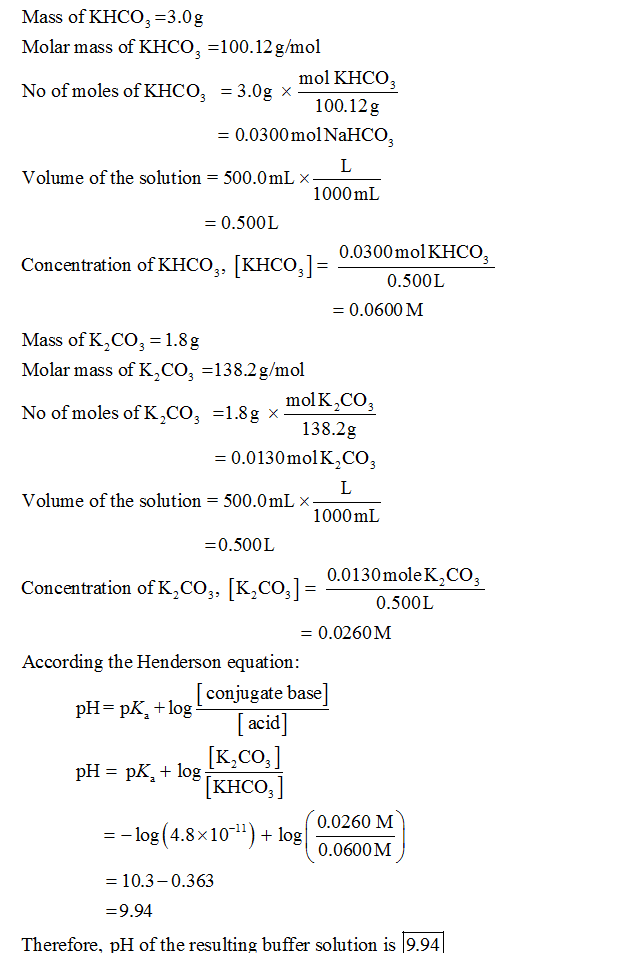In: Chemistry

# What is the pH of a solution made by dissolving 1.8 g of K2CO3 and 3.0...

What is the pH of a solution made by dissolving 1.8 g of K2CO3 and 3.0 g of KHCO3 in 500. mL of water? Use the Acid-Base Table. Please see Buffer pH for assistance.

## Solutions

##### Expert Solution## Related Solutions

##### Calculate the pH of the following buffer solutions: (a) a solution made by dissolving 4.969 g...
Calculate the pH of the following buffer solutions: (a) a solution made by dissolving 4.969 g KH2PO4 and 1.757 g K2HPO4 in water to give 200. mL of solution. Stepwise acid dissociation constants for phosphoric acid may be found in your textbook. (b) a solution made by mixing 10.00 mL 0.29 M (CH3)2NH with 40.00 mL 0.17 M (CH3)2NH2Cl. The base dissociation constant Kb is 7.40 x 10-4 for (CH3)2NH. Assume that the final volume is the sum of the...
##### 1- What is the pH of a solution made by dissolving 3.26 grams of calcium fluoride...
1- What is the pH of a solution made by dissolving 3.26 grams of calcium fluoride in enough water to make 3.0×102 mL of solution? The Ka for HF is 6.8x10–4. 2- The Kb of dimethylamine [(CH3)2NH] is 5.90x10–4 at 25°C. Calculate the pH of a 0.0930 M solution of dimethylamine.
##### What is the osmotic pressure of a solution made by dissolving 85.0 g of glucose, C6H12O6,...
What is the osmotic pressure of a solution made by dissolving 85.0 g of glucose, C6H12O6, in enough water to form 175.0 mL of solution at 17.0 ∘C ? Express your answer to three significant figures and include the appropriate units.
##### What is the osmotic pressure of a solution made by dissolving 75.0 g of glucose, C6H12O6,...
What is the osmotic pressure of a solution made by dissolving 75.0 g of glucose, C6H12O6, in enough water to form 250.0 mL of solution at 25.0 ∘C ?
##### A solution is made by a dissolving 16.8 g of sodium fluoride , NaF , in...
A solution is made by a dissolving 16.8 g of sodium fluoride , NaF , in enough water to make exactly 500.ml of solution . Calculate the molarity of each species: NaF, Na+ and F-.
##### A solution is made by dissolving 10.0 g of sodium chloride in 110.5 g of water...
A solution is made by dissolving 10.0 g of sodium chloride in 110.5 g of water at 25oC. The density of the solution is 1.021 g/mL 1.What is the osmotic pressure of this solution?    2.What is the boiling point of this solution?    3.What is the freezing point of this solution?
##### A solution is made by dissolving 0.571 mol of nonelectrolyte solute in 815 g of benzene....
A solution is made by dissolving 0.571 mol of nonelectrolyte solute in 815 g of benzene. Calculate the freezing point, Tf, and boiling point, Tb, of the solution.
##### A solution is made by dissolving 0.531 mol of nonelectrolyte solute in 765 g of benzene....
A solution is made by dissolving 0.531 mol of nonelectrolyte solute in 765 g of benzene. Calculate the freezing point, Tf, and boiling point, Tb, of the solution.
##### a) What is the molarity of the solution that was prepared by dissolving 3.25 g of...
a) What is the molarity of the solution that was prepared by dissolving 3.25 g of sulfuric acid in water to a total volume of 500.0 mL? b)What is the molarity of the hydrogen ion in part a if you assume the sulfuric acid ionizes completely? Write a balanced chemical equation.
##### A 0.100-L solution is made by dissolving 0.441 g of CaCl2(s) in water. (a) Calculate the...
A 0.100-L solution is made by dissolving 0.441 g of CaCl2(s) in water. (a) Calculate the osmotic pressure of this solution at 27 °C, assuming that it is completely dissociated into its component ions. (b) The measured osmotic pressure of this solution is 2.56 atm at 27 °C. Explain why it is less than the value calculated in (a), and calculate the van’t Hoff factor, i, for the solute in this solution. (c) The enthalpy of solution for CaCl2 is...